## 寫測試的流程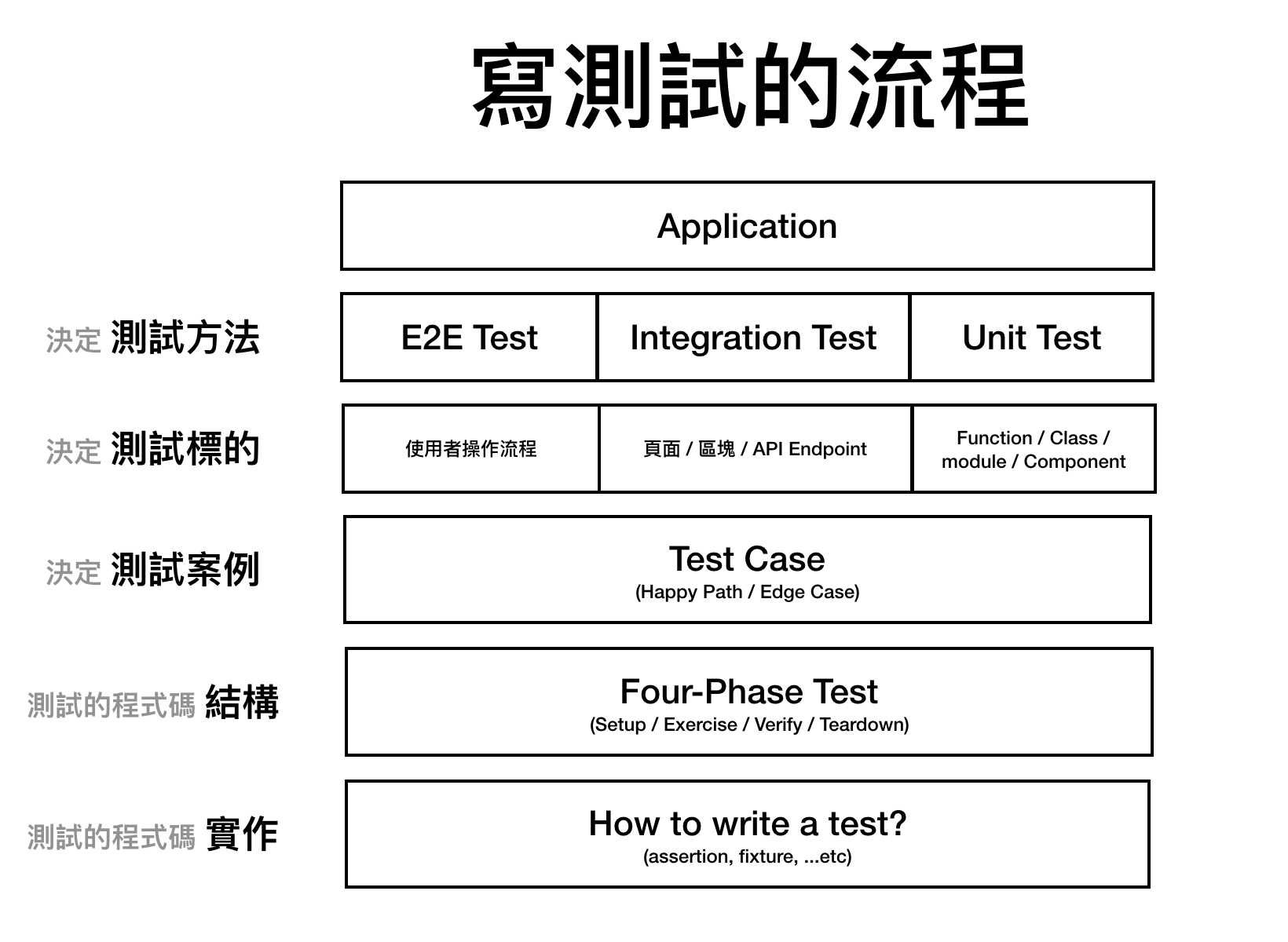### 決定測試方法

E2E Test 以使用者的角度來檢測系統是否可用，能夠測試到最多程式碼，但也因為涵蓋很多程式碼，不僅很容易壞掉，測試失敗的時候也難以判斷是系統的哪一部分出錯。

### 決定測試標的

E2E Test 的標的可以是使用者流程，以 Todo List 為例，可能的流程就有：

• 使用者新增一筆 Todo
• 使用者修改一筆 Todo
• 使用者刪除一筆 Todo
• 使用者清空 Todo

### 決定測試案例

``````function sum (a, b) {
return a + b
}
``````

Happy Path 為

• a 與 b 皆為數字的時候，會回傳兩者加總的整數

• 當沒有傳入 a 或 b
• 當 a 或 b 不為數字
• …促繁不及備載

• 測試的資料夾結構
• 測試檔案內如何排版程式碼
• 測試案例內的程式碼結構

## 測試的排版與結構

### 建立測試檔案

``````└── /some-folder
├── /__tests__
|   └── utils.test.js <--------- 測試檔案
└── utils.js          <--------- sum 函式所在檔案
``````

### 測試檔案內的排版

Jest 提供兩個 method `describe``test`

`describe` 來描述標的是什麼，`test` 描寫測試案例的預期結果

``````describe("#sum", () => { // <------- 描寫標的
test("return the sum of two numbers", () => { // <------- 描寫預期結果
// ... 執行測試
})
})
``````

``````describe("#sum", () => {
// `when receive two number` 描寫輸入
test("return the sum when receive two number", () => {

})

test("throw TypeError when receive other types instead of number", () => {

})
})
``````

``````describe("#sum", () => {
describe("when receive two number", () => {
test("return the sum", () => { })
test("return number", () => { })
})

describe("when receive undefined", () => {
test("throw TypeError", () => {

})

test("預期結果 1", () => {})
test("預期結果 2", () => {})
test("預期結果 3", () => {})
})
})
``````

## 一個測試案例的結構

``````test('我是一個測試案例', () => {
// 這裡會發生什麼事呢？？？
})
``````

### Phase 1 - Setup

``````const name = 'Jack'
const response = {
id: 5566,
name
}
const createUserMock = jest
// Mock - 建立假資料
.spyOn(api, 'createUser')
.mockImplementation(() => Promise.resolve(response))
``````

### Phase 2 - Exercise

``````const user = await User.create(name)
``````

### Phase 3 - Verify

``````expect(createUserMock).toHaveBeenCalledTimes(1)
expect(createUserMock).toHaveBeenCalledWith(name)
expect(user).toEqual(response)
``````

### Phase 4 - Teardown

``````createUserMock.mockRestore()
``````

### 結果

``````import * as api from '../apiWrapper'
import { create } from '../user'

test('User.create', async () => {
// Setup 建立測試資料與 Mock Stub
const name = 'Jack'
const response = {
id: 5566,
name
}
const createUserMock = jest
.spyOn(api, 'createUser')
.mockImplementation(() => Promise.resolve(response))

// Exercise 執行
const user = await User.create(name)

// Verify 驗證結果
expect(createUserMock).toHaveBeenCalledTimes(1)
expect(createUserMock).toHaveBeenCalledWith(name)
expect(user).toEqual(response)

// Teardown 消除 Mock，回到原始狀態
createUserMock.mockRestore()
})
``````

### 其他例子

``````test('#sum', () => {
// Setup 建立測試資料
const first = 3
const second = 4

// Exercise 執行待測目標
const result = sum(first, second)

// Verify 驗證結果
expect(result).toBe(7)

// Teardown
// 沒有
})
``````

## 合格的單元測試

Robert C. Martin，《無瑕的程式碼》一書提出單元測試應遵守 F.I.R.S.T 原則，我們可以依據該原則判斷單元測試是否合格。

### Independent

1. 如果測試程式碼相互依賴，那些依賴的部分我們也必須要視為待測函式的輸入，而那些輸入，在程式實際運行的環境是不存在的。
2. 當測試失敗時，無法很快的定位錯誤，因為測試之間相互關聯，測試 A 的失敗可能是 B 造成的。
3. 測試程式無法平行執行。

• 使用了全域變數。
• 測試案例之間共享變數，而沒有在每個測試案例前重置該變數。
• 沒有在每次測試案例執行之後將 mock 重置。

### Repeatable

1. 不同的瀏覽器
2. 執行測試時，不同機器使用不同的環境變數
3. 程式中使用了時間相關功能，像是當前日期或是隨機函式
4. 通過資料庫取得資料，而資料庫會被其他環境改變
5. 通過網路取得資料

1, 2 點我們可以通過一致的測試環境設定解決，而 3, 4, 5 我們需要透過 Mock 來消除那些變因

## 高效的單元測試

1. 寫盡可能少的測試（不能賺錢的程式少寫）
2. 無關的改動，不能讓測試壞掉（減少修測試所花的時間）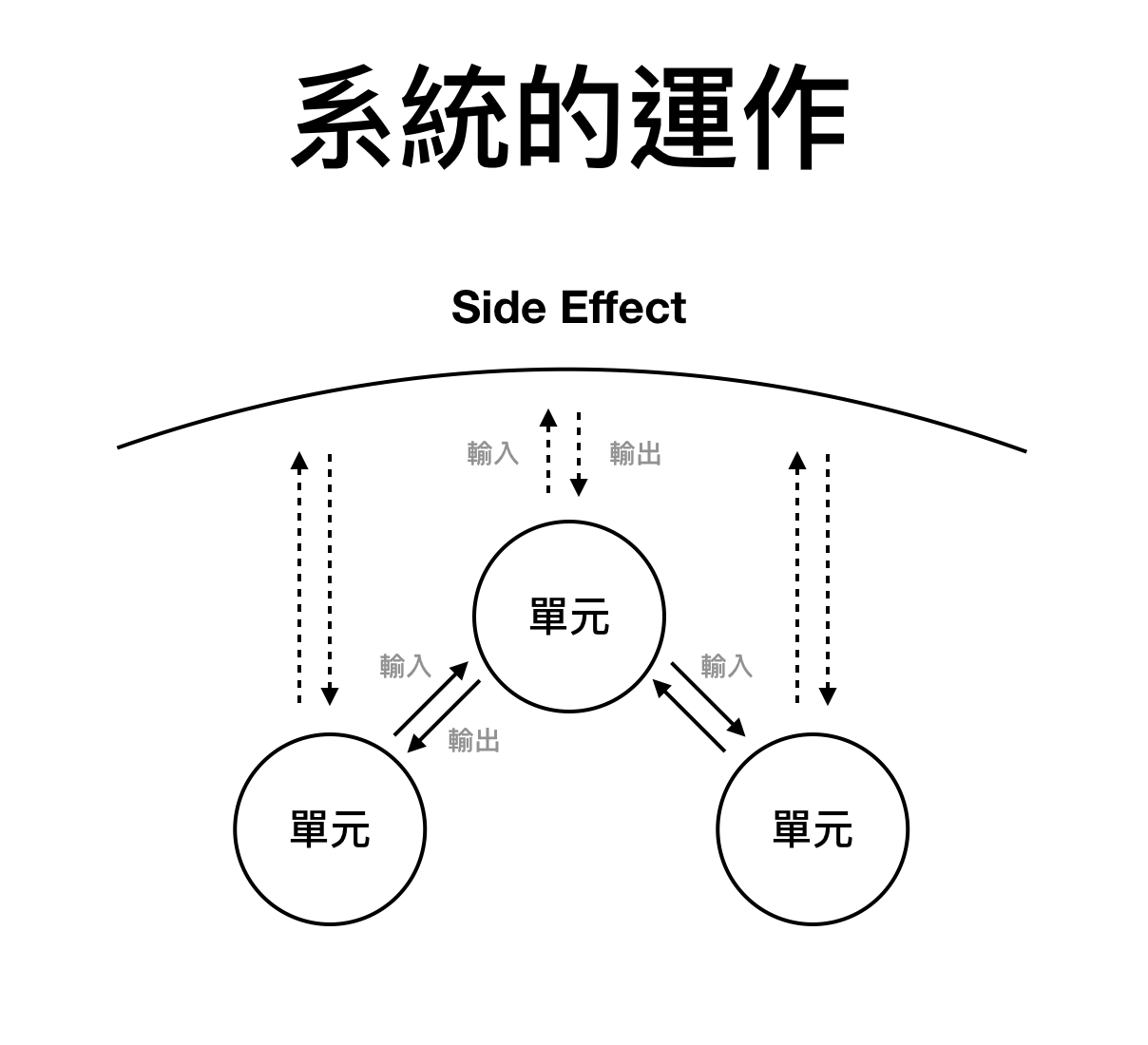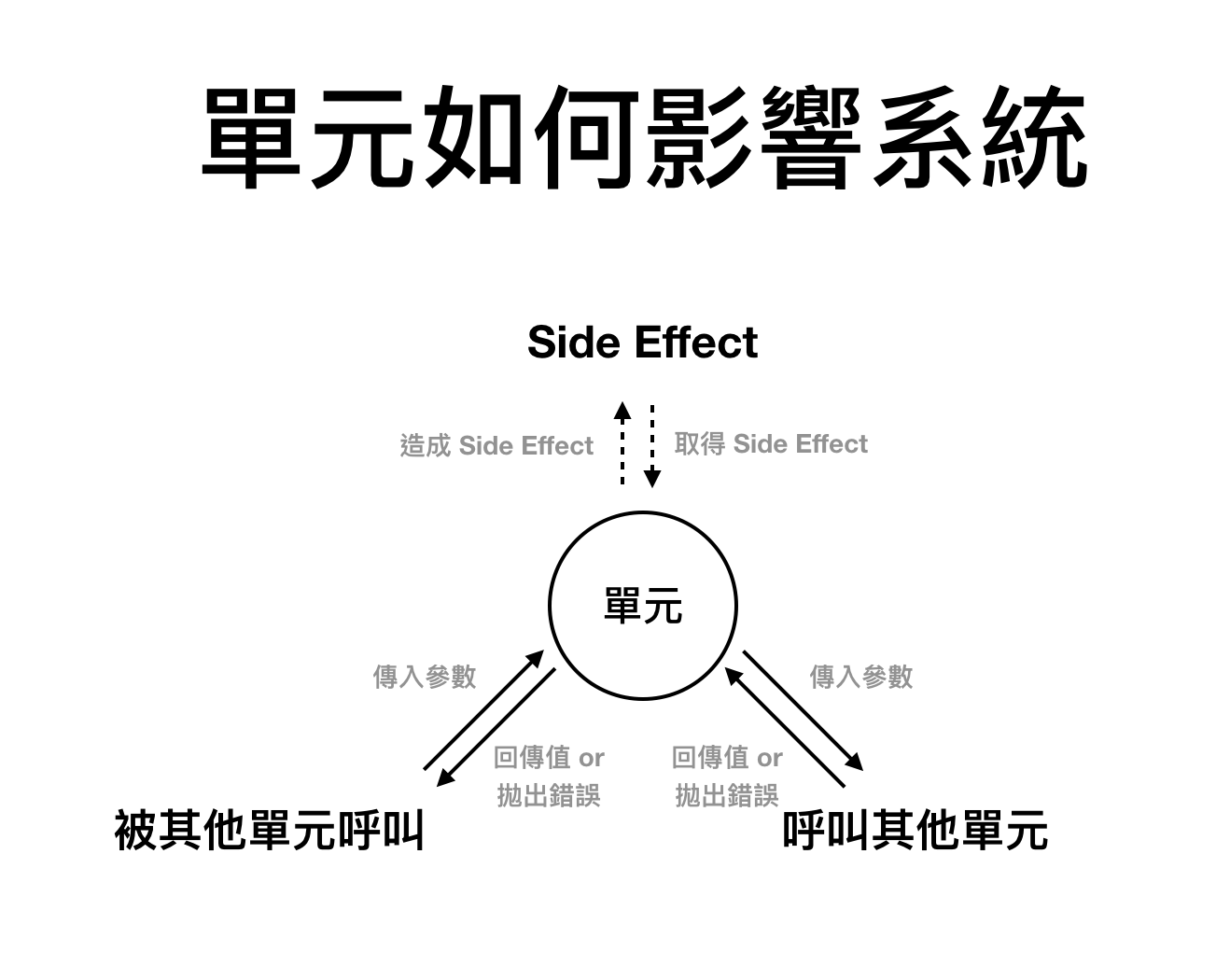Side Effect 指的是，除了兩個單元直接傳遞參數與回傳值之外，直接對外部的狀態造成影響，或是從外部的狀態取得資料。

### 被其他單元呼叫

4-phase 行為 範例程式 為什麼？
Setup 準備輸入的參數 `const a = 1; const b = 2`
Exercise 傳入參數
Verify 驗證回傳值是否如同預期 `expect(result).toBe(3)`
Teardown X

### 呼叫其他單元

#### 有 Side Effect，對外部狀態造成改變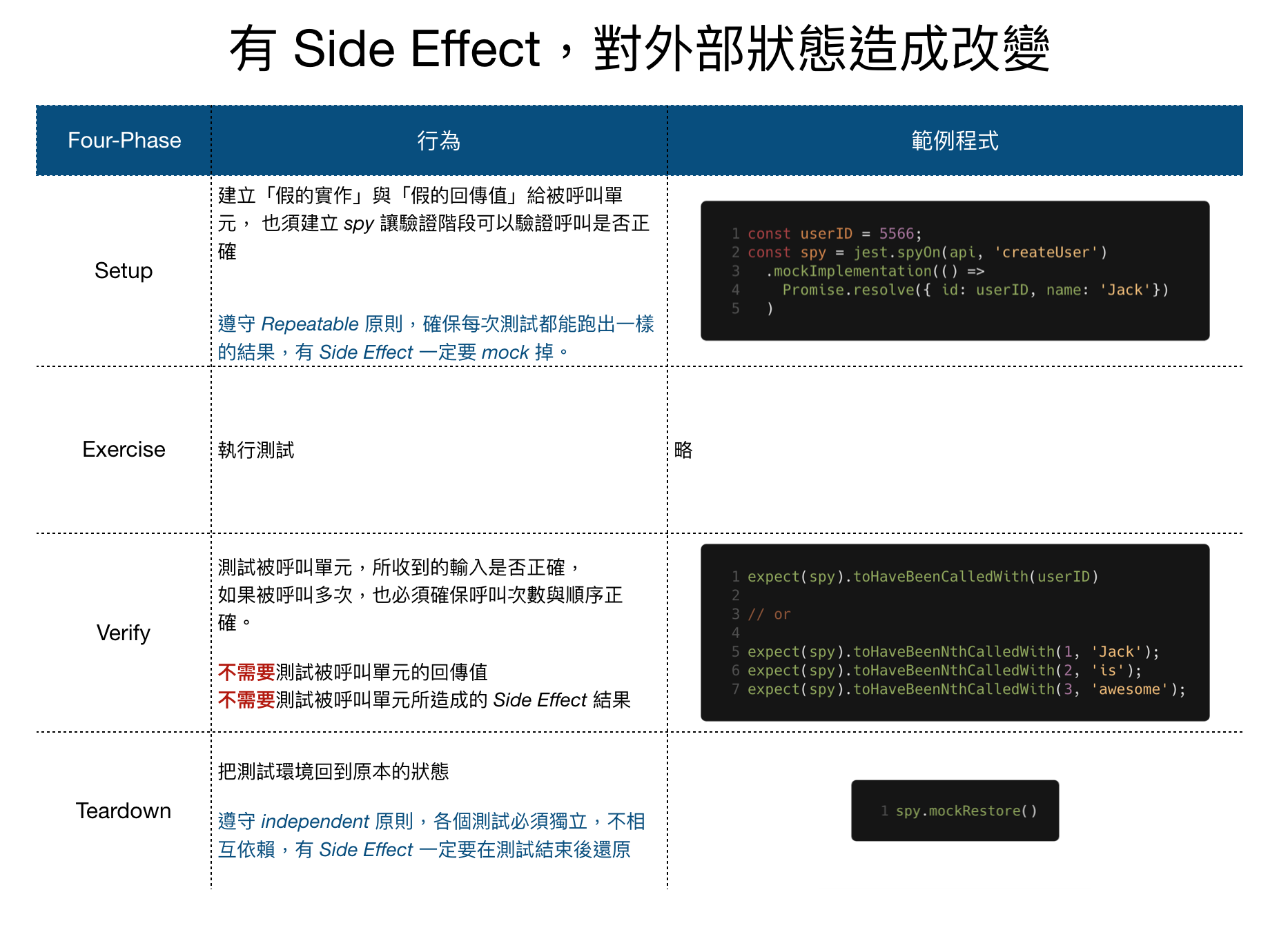#### 有 Side Effect，取得外部狀態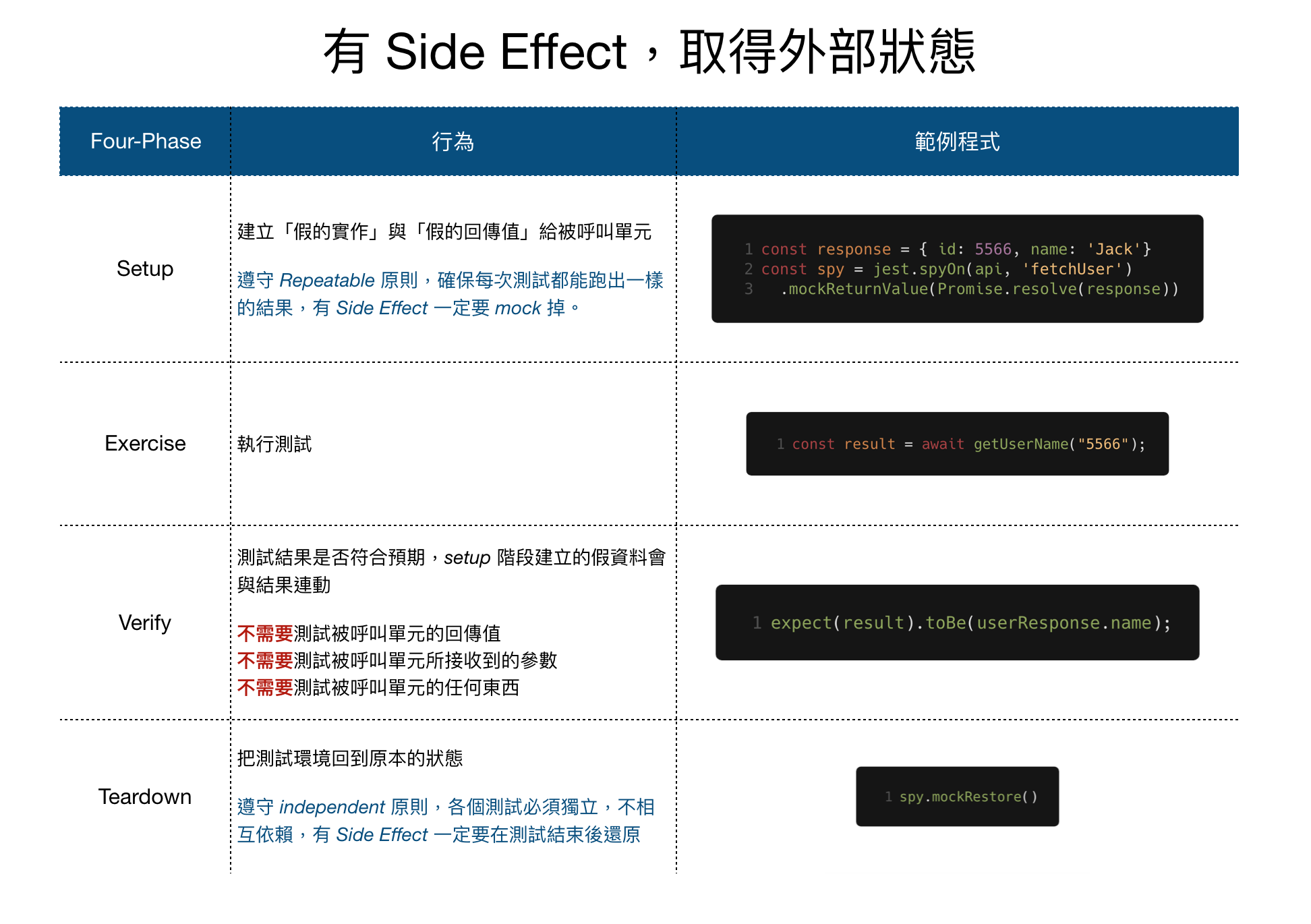## Jest 中 Expect 的使用

### 大部分的 Primitive Type

``````expect(5566).toBe(5566);
expect(true).toBe(true);
expect(null).toBe(null);
expect(undefined).toBe(undefined);
expect("Jack is awesome!").toBe("Jack is awesome!");
``````

### Float

Float 用 `toBeCloseTo` 就對了

``````expect(0.2 + 0.1).toBe(0.3);           // 💀 Failed
expect(0.2 + 0.1).toBeCloseTo(0.3, 5); // 👍 Correct
``````

### Object

``````expect({ a: "a", b: "b" }).toEqual({ a: "a", b: "b" }); // 👍
expect({ // nested 也 ok 👍
a: "a",
b: "b",
nested: { foo: "bar" }
}).toEqual({
a: "a",
b: "b",
nested: { foo: "bar" }
});
``````

``````expect({ a: "a", b: "b" }).toMatchObject({ a: "a", b: "b" }); // 👍
expect({ a: "a", b: "b" }).toMatchObject({ a: "a" }); // 👍
expect({ a: "a", b: "b" }).toMatchObject({ xxx: "xxx" }); // 💀 noooooooooooooooo
``````

### Array

``````expect([1, 2, { a: "a" }]).toEqual([1, 2, { a: "a" }]);
``````

``````expect([1, 2, { a: "a" }]).toContainEqual({ a: "a" });
``````

### 錯誤

``````test("Error", () => {
// 👍 預期錯誤的型別
expect(() => {
throw new TypeError("Jack is Awesome");
}).toThrow(TypeError);

// 👍 預期錯誤訊息的內容
expect(() => {
throw new TypeError("Jack is Awesome");
}).toThrow("Awesome");
});

``````

## Mock Method 與 Mock Module

1. 一但 mock 特定的 module，整份測試檔案都會生效，也不管某些測試案例不想要 mock 的行為
2. manual mock 被整個系統共用，沒辦法針對特出的場景設計 mock (待確認)
3. manual mock 要模擬真實的行為，通常會變成很複雜的實作，這種複雜度是我們希望在測試中避免的，畢竟我們不想再擔心程式之餘，還要擔心測試的 bug

``````// FileSummarizer.js
import fs from 'fs'

export function summarizeFilesInDirectorySync(directory) {
directory,
fileName,
}));
}
``````

``````// __tests__/FileSummarizer.test.js
import fs from 'fs'
import FileSummarizer from '../FileSummarizer'

describe('FileSummarizer#summarizeFilesInDirectorySync', () => {
const files = ['jack.js', 'is.js', 'awesome.avi']
let spy
beforeEach(() => {
// setup - 作假
});

afterEach(() => {
// teardown - 清空 side effect
spy.mockRestore()
})

test('includes all files in the directory in the summary', () => {
const directory = '/path/to'

// exercise
const fileSummary = FileSummarizer.summarizeFilesInDirectorySync(directory);

// verify
expect(fileSummary).toEqual([
{
directory,
fileName: files
},
{
directory,
fileName: files
},
{
directory,
fileName: files
},
]);
});
});
``````

### Test Helper

``````beforeEach(() => {
});
``````

``````// ../test/fsHelpers.js
import fs from 'fs';

export function spyFs(moethodName, returnedValue) {
return jest.spyOn(fs, methodName).mockReturnValue(returnedValue);
}

// original test file
import { spyFs } from '../test/fsHelper'

beforeEach(() => {
});
``````

### 使用 mock Module 的情境

1. 沒有使用 dependency injection
2. 該 module 的不同的 methods 在同一個測試案例中被使用，且 module 內部會共享狀態
3. module export default 一個 function，在測試被使用